﻿ 一种适合迭代求解的反馈力浸入边界法Download PDF文章快速检索 高级检索

Feedback forcing immersed boundary method for iterative calculations
LI Xu, ZHOU Zhou, XUE Chen
School of Aeronautics, Northwestern Polytechnical University, Xi'an 710072, China
Abstract: This paper proposes a novel idea of Goldstein's virtual boundary method which improves the calculation of the feedback forcing term and extends the applicability of this immersed boundary method. The original virtual boundary method includes the time integration of velocity deviation, therefore confining this method to time-dependent Navier-Stokes (N-S)equations with a severe limitation of time steps for the explicit scheme. In contrast, this paper calculates the feedback forcing by the sum of velocity deviation in iteration to avoid time dependent parameters. Thus, the improved method is not only suitable for the unsteady implicit scheme, but can couple with the steady solver without any time-dependent terms. To verify this improved method, this paper simulated the flow past a stationary cylinder, the inline oscillation of a cylinder in a fluid at rest, a flapping ellipse wing and a stationary sphere. All results agree well with previous numerical results, verifying the accuracy of the present method. We come to the conclusions that the feedback force is dependent on the velocity deviation during iteration, and that the present method can couple with the implicit algorithm for unsteady flows as well as the steady Navier-Stokes solver, indicating wider applicability of the present method for extensive flow problems.
Keywords: immersed boundary method    feedback forcing term    implicit scheme    orthogonal grid    moving boundary

1 数值计算方法 1.1 控制方程

 $\left\{ {\begin{array}{*{20}{l}} {\rho \left( {\frac{{\partial \mathit{\boldsymbol{u}}}}{{\partial t}} + \mathit{\boldsymbol{u}} \cdot \boldsymbol{\nabla} \mathit{\boldsymbol{u}}} \right) + \boldsymbol{\nabla} p = \mu {\boldsymbol{\nabla} ^2}\mathit{\boldsymbol{u}} + \mathit{\boldsymbol{F}}(x, t)}\\ {\boldsymbol{\nabla} \cdot \mathit{\boldsymbol{u}} = {\bf{0}}} \end{array}} \right.$ （1）

 $\mathit{\boldsymbol{F}}(x, t) = - \int \mathit{\boldsymbol{f}} \left( {{x_{\rm{s}}}, t} \right)\delta \left( {x - {x_{\rm{s}}}} \right){\rm{d}}{x_{\rm{s}}}$ （2）

 $\begin{array}{*{20}{c}} {\mathit{\boldsymbol{f}}\left( {{x_{\rm{s}}}, t} \right) = \alpha '\int_0^t {\left( {\mathit{\boldsymbol{u}}(x, t) - \mathit{\boldsymbol{u}}\left( {{x_{\rm{s}}}, t} \right)} \right)} {\rm{d}}t + }\\ {\beta '\left( {\mathit{\boldsymbol{u}}(x, t) - \mathit{\boldsymbol{u}}\left( {{x_{\rm{s}}}, t} \right)} \right)} \end{array}$ （3）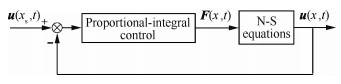图 1 反馈力浸入边界法原理 Fig. 1 Principle of feedback forcing immersed boundary method

Delta函数是物面节点与流场网格单元信息交流的关键，其形式并不唯一，本文选用的Delta函数为

 $\begin{array}{l} \delta (r) = \\ \left\{ \begin{array}{l} \frac{1}{8}\left( {3 - 2|r| + \sqrt {1 + 4|r| - 4{r^2}} } \right)\;\;\;\;\;\;\;\;0 \le |r| < 1\\ \frac{1}{8}\left( {5 - 2|r| - \sqrt { - 7 + 12|r| - 4{r^2}} } \right)\;\;\;\;1 \le |r| < 2\\ 0\;\;\;\;\;\;\;\;\;\;\;\;\;\;\;\;\;\;\;\;\;\;\;\;\;\;\;\;\;\;\;\;\;\;\;\;\;\;\;\;\;\;\;\;\;\;\;\;\;\;\;|r| \ge 2 \end{array} \right. \end{array}$ （4）

 ${N_x} = \int_{\partial S} {{f_x}} {\rm{d}}s + \rho \frac{{{\rm{d}}{u_{{\rm{c}}, x}}}}{{{\rm{d}}t}}V$ （5）

1.2 反馈力浸入边界法的改进

Goldstein的反馈力浸入边界法(后文统称原始方法)与显式求解方法结合将严重限制计算的效率，难以在工程应用中推广。而隐式格式通常没有稳定性的限制，将反馈力浸入边界法与隐式格式结合，是一种有效的解决办法，也能更好地发挥反馈力浸入边界法的优点，这正是本文改进方法的出发点。

 $\begin{array}{l} \mathit{\boldsymbol{f}}\left( {{x_{\rm{s}}}, t} \right) = \alpha '\sum\limits_{i = 1}^{N'} {\left( {\mathit{\boldsymbol{u}}(x, \Delta t \cdot i) - \mathit{\boldsymbol{u}}\left( {{x_{\rm{s}}}, \Delta t \cdot i} \right)} \right)} \Delta t + \\ \;\;\;\;\;\;\beta '\left( {\mathit{\boldsymbol{u}}(x, t) - \mathit{\boldsymbol{u}}\left( {{x_{\rm{s}}}, t} \right)} \right) \end{array}$ （6）

 $\begin{array}{l} \mathit{\boldsymbol{f}}\left( {{x_{\rm{s}}}, N} \right) = \alpha \sum\limits_{i = 1}^N {\left( {\mathit{\boldsymbol{u}}\left( {x, i} \right) - \mathit{\boldsymbol{u}}\left( {{x_{\rm{s}}}, i} \right)} \right)} + \\ \;\;\;\;\;\beta \left( {\mathit{\boldsymbol{u}}(x, N) - \mathit{\boldsymbol{u}}\left( {{x_{\rm{s}}}, N} \right)} \right) \end{array}$ （7）

Fluent是目前一款应用较广泛的CFD商业软件，其基于有限体积法，求解器分为两大类：密度基和压力基。密度基求解器定常与非定常计算均按照时间推进求解，压力基求解器则不同。对于定常计算，压力基求解器求解的是不含时间项的N-S方程，非定常计算才含有时间项，且为隐式时间推进。本文选用压力基求解器，基于软件中的用户自定义函数(User-Defined Functions，UDFs)，通过一系列定常与非定常算例的计算，验证本文改进反馈力浸入边界法的有效性。

2 改进反馈力浸入边界法的验证 2.1 静止圆柱绕流

 ${C_D} = \frac{{{N_x}}}{{0.5\rho U_\infty ^2D}}$ （8）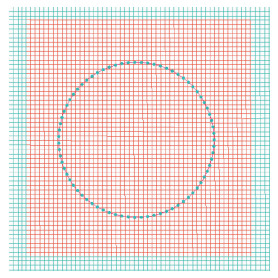图 2 计算网格分布 Fig. 2 Grid distribution in simulation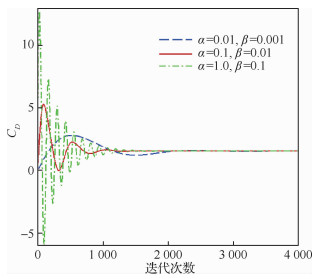图 3 反馈力参数对收敛的影响 Fig. 3 Influence of feedback forcing parameters for iteration

 方法 CD L/D 文献 1.58 2.35 文献 1.54 2.36 文献 1.565 2.31 本文Simplec 1.56 2.36 本文Couple 1.56 2.36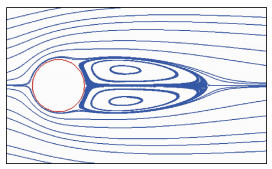图 4 圆柱流线(Re=40) Fig. 4 Streamlines on circular cylinder at Re=40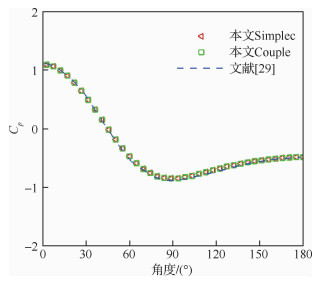图 5 静止圆柱压力系数 Fig. 5 Pressure coefficient for stationary circular cylinder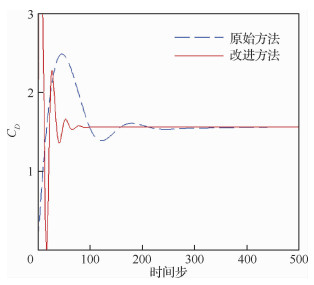图 6 静止边界隐式计算收敛效率对比 Fig. 6 Comparison of efficiency for implicit iterations at stationary boundary

 ${\rm{CFL}} = \frac{{{U_\infty }\Delta t}}{{\Delta x}}$

 方法 CFL CD 本文方法 3 946 1.56 文献 0.2 1.66

2.2 振荡圆柱

 $x(t) = - A\sin (2{\rm{ \mathit{ π} }}ft)$ （9）

 $Re = \frac{{{\rho _\infty }{u_{\max }}D}}{\mu }, {\rm{KC}} = \frac{{{u_{\max }}}}{{fD}}$ （10）

 算例名称 α β 静止圆柱 0.1 0.01 振荡圆柱 0.1 0.01 运动椭圆翼 0.2 0.02 静止圆球 50 0.1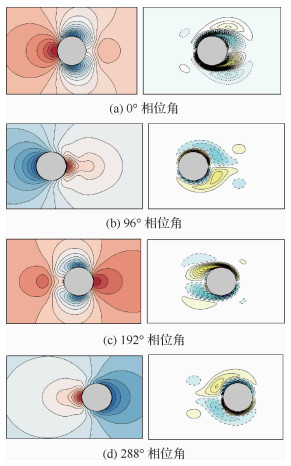图 7 不同相位下的涡量/压力等值线 Fig. 7 Vorticity/pressure contours at different phase angles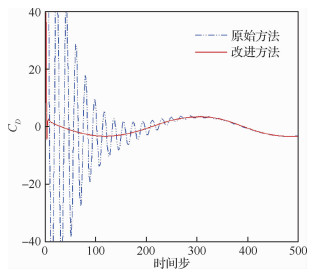图 8 运动边界隐式计算效率对比 Fig. 8 Comparison of efficiency for implicit iterations at moving boundary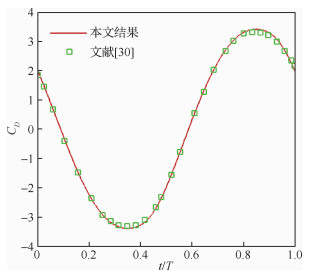图 9 阻力系数变化曲线 Fig. 9 Time history of drag coefficient
2.3 运动椭圆翼

 ${A(t) = \frac{{{A_0}}}{2}\left[ {\cos \left( {\frac{{2t}}{{{A_0}}}} \right) + 1} \right]}$ （11）
 ${\theta (t) = {\theta _0}\left[ {1 - \sin \left( {\frac{{2t}}{{{A_0}}}} \right)} \right]}$ （12）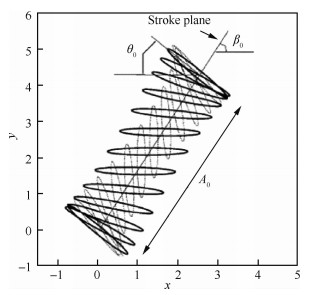图 10 椭圆翼运动路径 Fig. 10 Flapping path of ellipse wing

 ${C_D} = \frac{{{N_x}}}{{0.5cu_{\max }^2}}, {C_L} = \frac{{{N_y}}}{{0.5cu_{\max }^2}}$ （13）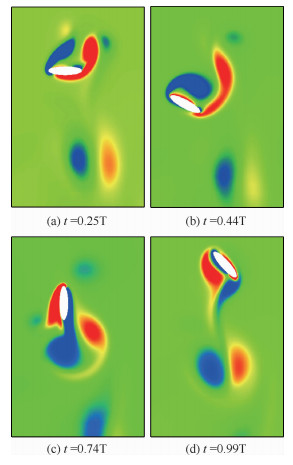图 11 一个周期内不同时刻的涡量图 Fig. 11 Vorticity fields during one period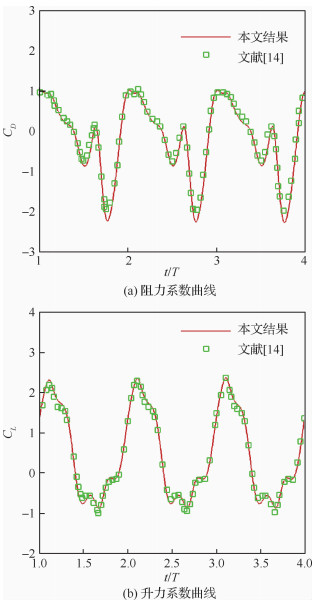图 12 扑动过程中的气动力曲线 Fig. 12 Curves of aerodynamic coefficients during flapping
2.4 静止圆球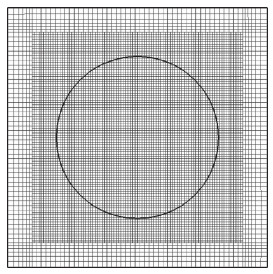图 13 局部网格加密 Fig. 13 Local refining for computation mesh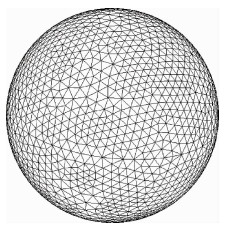图 14 圆球表面的Lagrange网格单元 Fig. 14 Lagrange mesh of sphere surface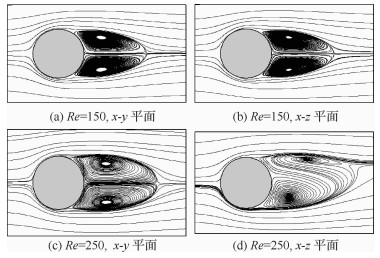图 15 不同雷诺数圆球绕流流线 Fig. 15 Streamlines for flow past sphere at different Re

 雷诺数 方法 CD Cc 150 文献 0.90 文献 0.91 本文结果 0.90 250 文献 0.70 0.061 文献 0.722 本文结果 0.717 0.061
3 结论

1) 可直接基于迭代次数对反馈力源项进行计算。与原始方法相比，由于不含时间相关参数，改进的反馈力浸入边界法可与求解定常N-S方程的松弛迭代方法结合，用于定常流动计算。

2) 隐式时间推进可以避免反馈力浸入边界法显式求解时严格的时间步长限制。本文改进的反馈力浸入边界法适合于隐式时间推进，可实现对非定常N-S方程的求解，且收敛特性要优于原始方法。

3) 改进的方法与原始方法均是基于速度误差反馈的思想求解力源项，但本文改进的反馈力浸入边界法有更广泛的适用性。

  NOACK R W, BOGER D A, KUNZ R F, et al. Suggar++: An improved general overset grid assembly capability[C]//19th AIAA Computational Fluid Dynamics. Reston: AIAA, 2009.  PESKIN C S. Numerical analysis of blood flow in the heart[J]. Journal of Computational Physics, 1977, 25(3): 220-252. Click to display the text  MITTAL R, IACCARINO G. Immersed boundary methods[J]. Annual Review of Fluid Mechanics, 2005, 37: 239-261. Click to display the text  SOTIROPOULO F, YANG X L. Immersed boundary methods for simulating fluid-structure interaction[J]. Progress in Aerospace Sciences, 2014, 65: 1-21. Click to display the text  KIM W, CHOI H. Immersed boundary methods for fluid-structure interaction:A review[J]. International Journal of Heat and Fluid Flow, 2019, 75(1): 301-309. Click to display the text  GOLDSTEIN D, HANDER R, SIROVICH L. Modeling a no-slip flow boundary with an external force field[J]. Journal of Computational Physics, 1993, 105(2): 354-366. Click to display the text  SAIKI E M, BIRINGEN S. Numerical simulation of a cylinder in uniform flow:Application of a virtual boundary method[J]. Journal of Computational Physics, 1996, 123(2): 450-465. Click to display the text  李秋实, 徐飞, 李志平. 一种包含运动边界的高精度流场数值计算方法[J]. 航空学报, 2014, 35(7): 1815-1824. LI Q S, XU F, LI Z P. A numerical method for simulating flow involving moving boundaries with high order accuracy[J]. Acta Aeronautica et Astronautica Sinica, 2014, 35(7): 1815-1824. (in Chinese) Cited By in Cnki (4) | Click to display the text  WANG Z, DU L, ZHAO J S, et al. Structural response and energy extraction of a fully passive flapping foil[J]. Journal of Fluids and Structures, 2017, 72: 96-113. Click to display the text  LI X J, ZHAO R G, ZHONG C W. Novel immersed boundary-lattice Boltzmann method based on feedback law[J]. Transactions of Nanjing University of Aeronautics and Astronautics, 2012, 29(2): 179-186. Click to display the text  HUANG W X, SHIN S J, SUNG H J. Simulation of flexible filaments in a uniform flow by the immersed boundary method[J]. Journal of Computational Physics, 2007, 226(2): 2206-2228. Click to display the text  SHOELE K, ZHU Q. Leading edge strengthening and the propulsion performance of flexible ray fins[J]. Journal of Fluid Mechanics, 2012, 693: 402-432. Click to display the text  GONG C L, HAN J K, YUAN Z J, et al. Numerical investigation of the effects of different parameters on the thrust performance of three dimensional flapping wings[J]. Aerospace Science and Technology, 2018, 84: 431-445. Click to display the text  XU S, WANG Z J. An immersed interface method for simulating the interaction of a fluid with moving boundaries[J]. Journal of Computational Physics, 2006, 216(2): 454-493. Click to display the text  HAN J K, ZHANG Y, CHEN G. Effects of individual horizontal distance on the three-dimensional bionic flapping multi-wings in different schooling configurations[J]. Physics of Fluids, 2019, 31(4): 1903-1919. Click to display the text  胡国暾, 杜林, 孙晓峰. 基于浸入式边界法的叶栅颤振数值模拟[J]. 航空学报, 2015, 36(7): 2269-2278. HU G D, DU L, SUN X F. Numerical simulation of an oscillating cascade based on immersed boundary method[J]. Acta Aeronautica et Astronautica Sinica, 2015, 36(7): 2269-2278. (in Chinese) Cited By in Cnki (2) | Click to display the text  ZHONG G H, DU L, SUN X F. Numerical investigation of an oscillating airfoil using immersed boundary method[J]. Journal of Thermal Science, 2011, 20(5): 413-422. Click to display the text  DU L, SUN X F, VIGOR Y. Generation of vortex lift through reduction of rotor/stator gap in turbo machinery[J]. Journal of Propulsion and Power, 2015, 32(2): 1-14. Click to display the text  WANG L, CURRAO G, HAN F, et al. An immersed boundary method for fluid-structure interaction with compressible multiphase flows[J]. Journal of Computational Physics, 2017, 346: 131-151. Click to display the text  GUO X X, YAO J K, ZHONG C W, et al. A hybrid adaptive-gridding immersed-boundary lattice Boltzmann method for viscous flow simulations[J]. Applied Mathematics and Computation, 2015, 267(9): 529-553. Click to display the text  YANG X L, ZHANG X, LI Z L, et al. A smoothing technique for discrete delta functions with application to immersed boundary method in moving boundary simulations[J]. Journal of Computational Physics, 2009, 228(20): 7821-7836. Click to display the text  LEE C. Stability characteristics of the virtual boundary method in three-dimensional applications[J]. Journal of Computational Physics, 2003, 184(2): 559-591. Click to display the text  SHIN S J, HUANG W X, SUNG H J. Assessment of regularized delta functions and feedback forcing schemes for an immersed boundary method[J]. International Journal for Numerical Methods in Fluids, 2008, 58(3): 263-286. Click to display the text  ANSYS FLUENT. Release 14.0, theory guide[M]. Ansys Inc, 2011.  袁瑞峰.气体动理论格式的浸入边界法研究[D].西安: 西北工业大学, 2015. YUAN R F. The immersed-boundary method for gas-kinetic scheme[D]. Xi'an: Northwestern Polytechnical University, 2015(in Chinese).  HAMA R, MELDI M, FAVIER J, et al. A pressure-corrected immersed boundary method for the numerical simulation of compressible flows[J]. Journal of Computational Physics, 2018, 374: 361-383. Click to display the text  WANG S Z, ZHANG X. An immersed boundary method based on discrete stream function formulation for two- and three-dimensional incompressible flows[J]. Journal of Computational Physics, 2011, 230(9): 3479-3499. Click to display the text  WU J, SHU C. Implicit velocity correction-based immersed boundary-lattice Boltzmann method and its applications[J]. Journal of Computational Physics, 2010, 228(6): 1963-1979. Click to display the text  LEE D S, HA M Y, KIM S J, et al. Application of immersed boundary method for flow over stationary and oscillating cylinders[J]. Journal of Mechanical Science and Technology, 2006, 20(6): 849-863. Click to display the text  DÜTSCH H, DURST F, BECKER S, et al. Low-Reynolds-number flow around an oscillating circular cylinder at low Keulegan-Carpenter numbers[J]. Journal of Fluid Mechanics, 1998, 360: 249-271. Click to display the text  WANG Z J. Computation of insect hovering[J]. Mathematical Methods in the Applied Sciences, 2001, 24: 1515-1521. Click to display the text  JOHNSON T A, PATEL V C. Flow past a sphere up to a Reynolds number of 300[J]. Journal of Fluid Mechanics, 1999, 378: 19-70. Click to display the text  WANG Y, SHU C, YANG L M, et al. An immersed boundary-lattice Boltzmann flux solver in a moving frame to study three-dimensional freely falling rigid bodies[J]. Journal of Fluids and Structures, 2017, 68: 444-465. Click to display the text  WANG Y, SHU C, TEO C J, et al. An efficient immersed boundary-lattice Boltzmann flux solver for simulation of 3D incompressible flows with complex geometry[J]. Computers & Fluids, 2016, 124: 54-66. Click to display the text
http://dx.doi.org/10.7527/S1000-6893.2020.23712

0

#### 文章信息

LI Xu, ZHOU Zhou, XUE Chen

Feedback forcing immersed boundary method for iterative calculations

Acta Aeronautica et Astronautica Sinica, 2020, 41(9): 123712.
http://dx.doi.org/10.7527/S1000-6893.2020.23712